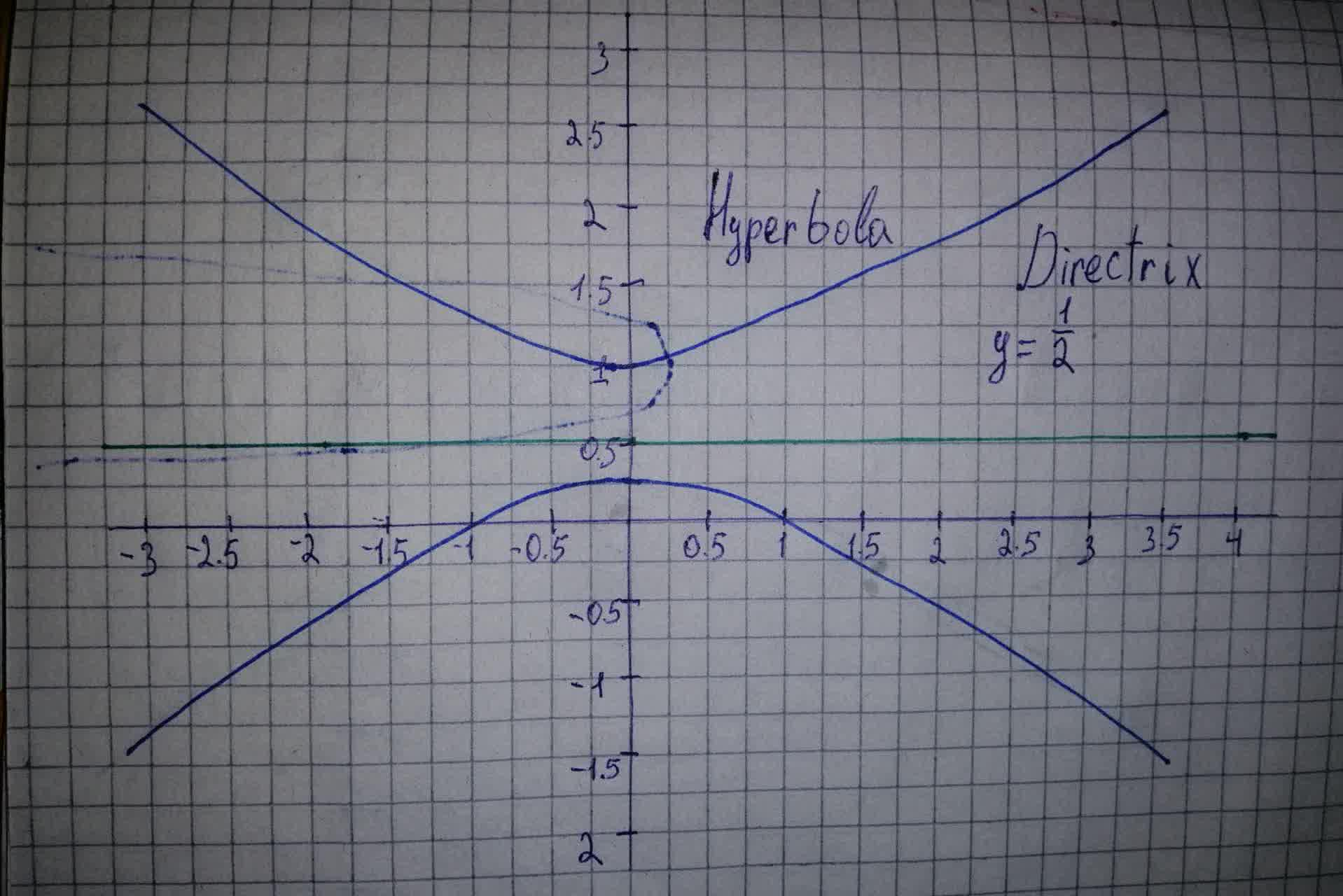# Graph the lines and conic sections r=\frac{1}{(1+2\sin\theta)}Dottie Parra 2021-08-09 Answered
Graph the lines and conic sections $r=\frac{1}{\left(1+2\mathrm{sin}\theta \right)}$
You can still ask an expert for help

• Questions are typically answered in as fast as 30 minutes

Solve your problem for the price of one coffee

• Math expert for every subject
• Pay only if we can solve itjoshyoung05M
Step 1
Consider the given polar equation,
$r=\frac{1}{\left(1+2\mathrm{sin}\left(\theta \right)\right)}$
Compare the given with standard equation of the hyperbola,
$r=\frac{ke}{1+2\mathrm{sin}\left(\theta \right)}$
we get,
$e=2$ and $k=\frac{1}{2}$
so the given equation is the equation of the hyperbola.
Step 2
So, the graph of the hyperbola is,